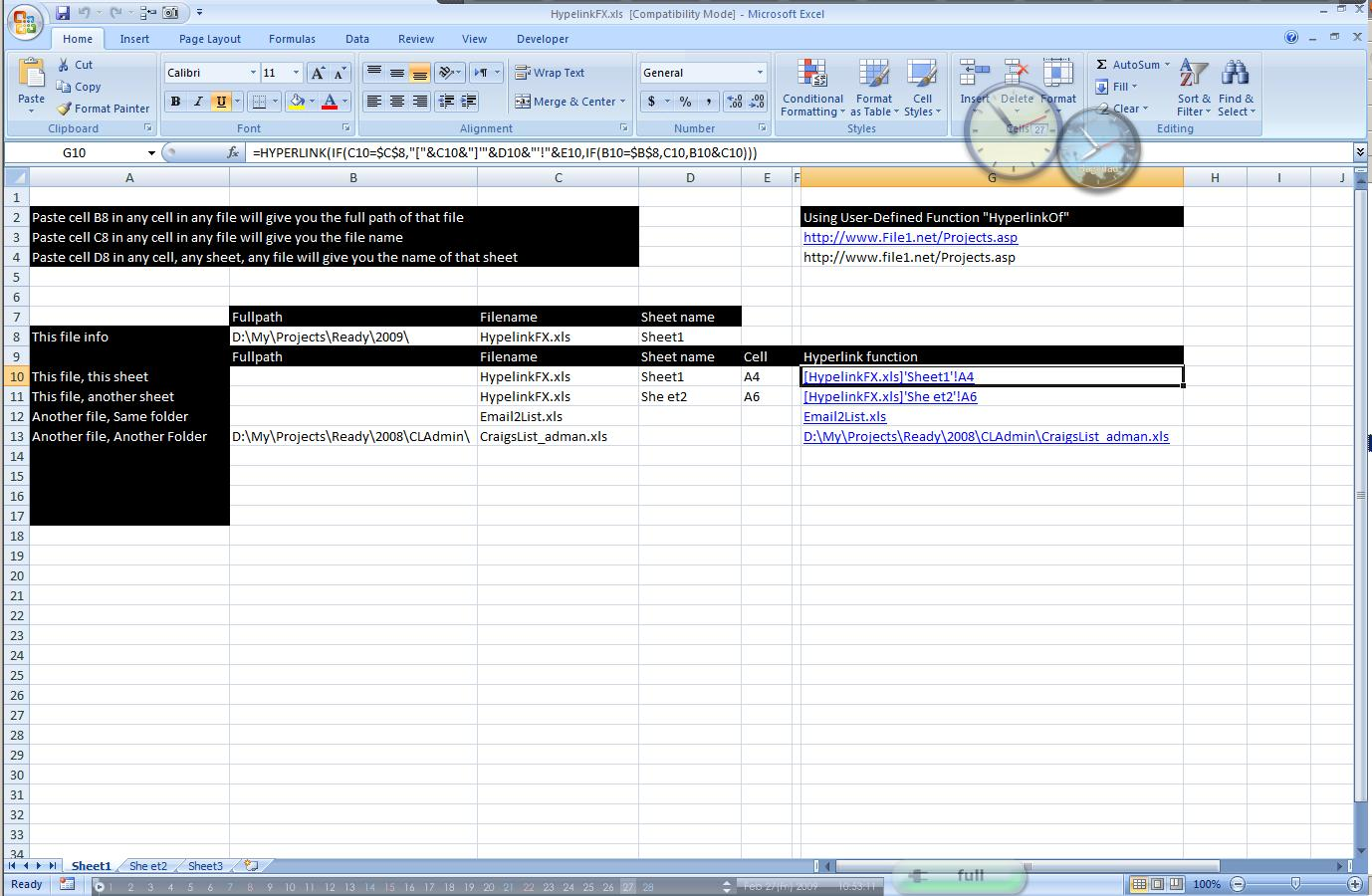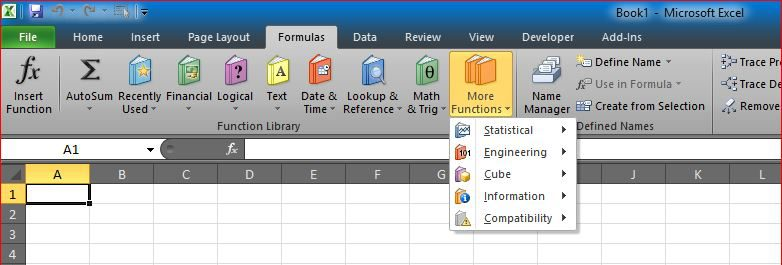## Tally chart in functions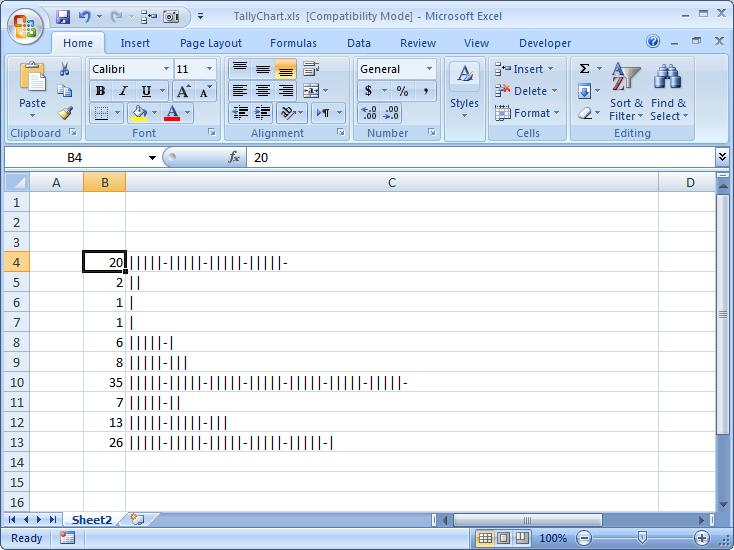Using a single function can make a graphical view to your number Function is REPT along with IF and INT to show that number in graphical view, formula used here …

## CamelCase to Camel Case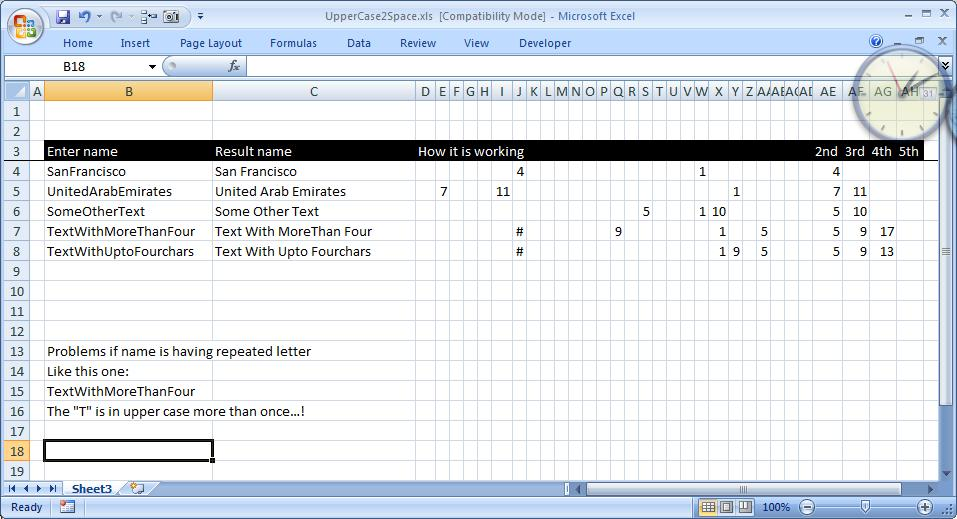Here is a good practice for combining functions to get a full smartsheet It basically add a space before any capital letter in a text, helpful when you have strings …

## Sum of divide – Array formula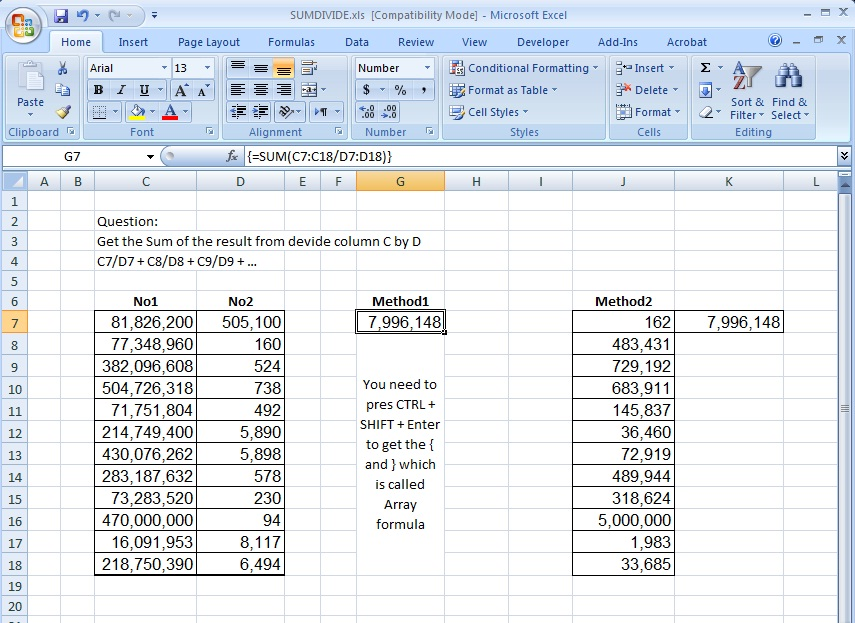This is the answer to an old question: Here is the story behind it: I had a client who calls me every now and then asking the same question: ” …

## Standard math to calculate Aspect Ratio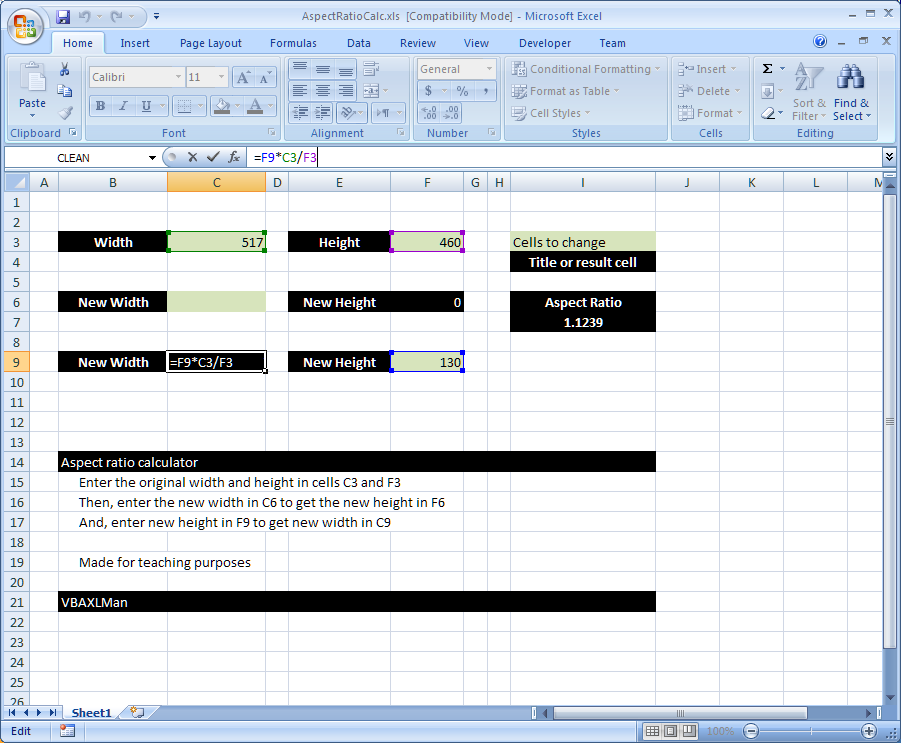Here is a simple formula that uses standard math functions (+ , – , / and *) to calculate the aspect ratio I often need some simple formula like this …

## MID + SEARCH to convert cell to rows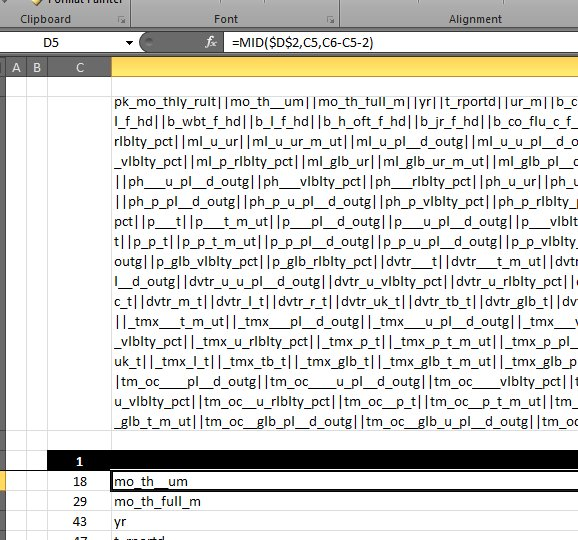I have been there and done that more than once before This time I had a really large cell, more than 3k characters need to be converted into rows, and …